# Plotting Von Misses Stress X Distance or Coordinate Node or Element

I would like to plot a graph with the edge von misses stress, like the picutre below. But in place of time, I would like to plot the X coordinate along the edge. So the results will be a line per time like the second picture. Is there any command in apdl that do it??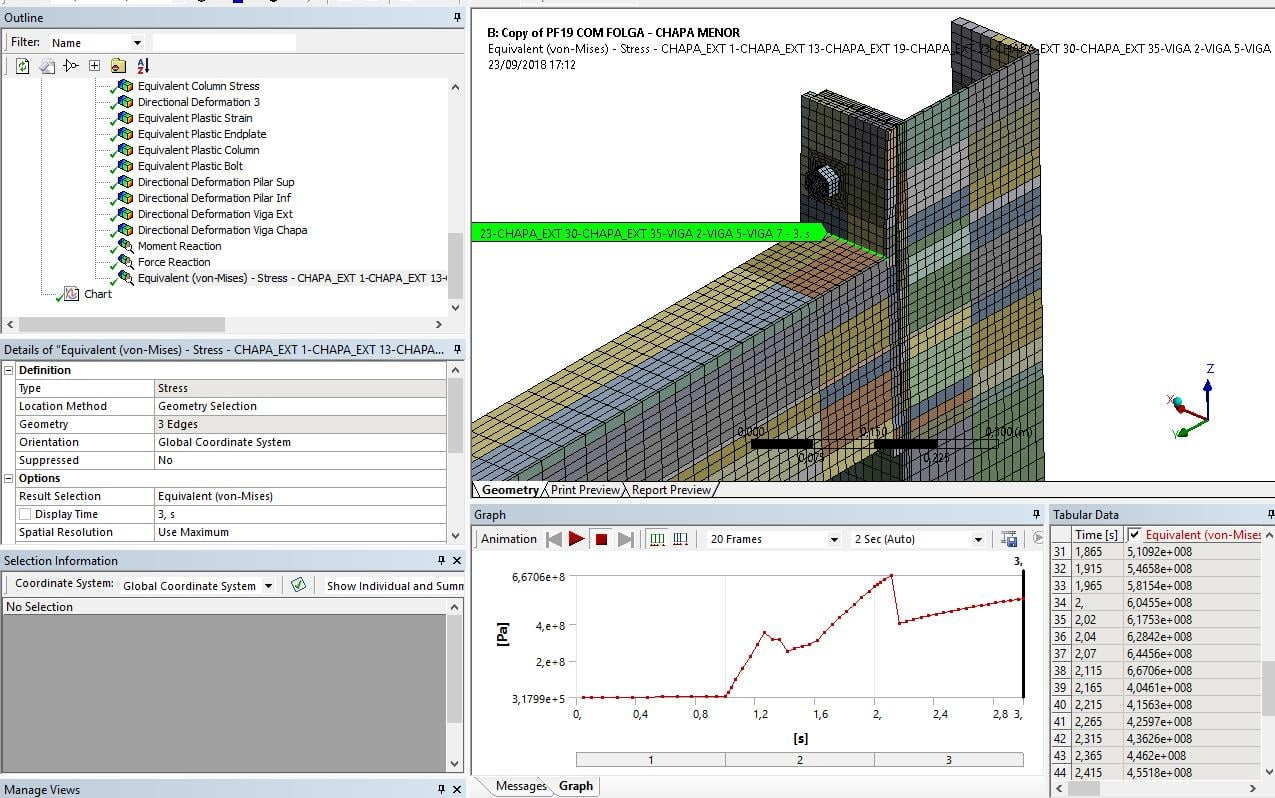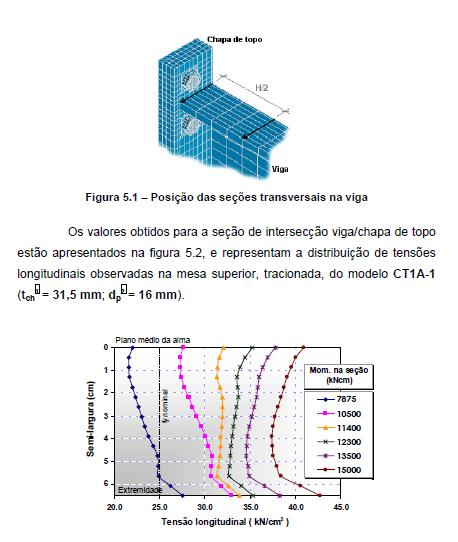• Fabricio,

In Mechanical, you use Construction Geometry to create a Path to plot stress along that path.  Here is the SimuTech group tutorial.

Regards,

Peter

• edited September 2018

Hello Fabricio,

As Peter suggested, you can get distance along the edge vs stress plot using Construction Geometry (Path).

If you want to have X-coordinate (and not distance along the edge) vs stress plot instead, you can create in the following way.

1. Create a Equivalent stress result along a path as Peter suggested

2. Create a User defined result along a path, with expression LOCX

3. Highlight both objects created above and create a Chart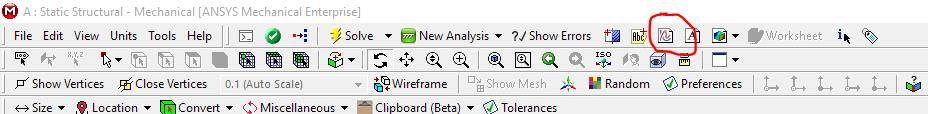4. In the created chart, change the X axis (under Chart Controls) from "Length" to this "User defined result" created in step 2 to get  X-coordinate vs stress plot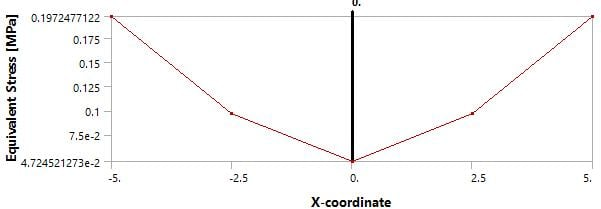You can also combine multiple Equivalent stress results (at multiple time points) into one chart.

Hope this helps.

Best regards,

Rohith

• edited September 2018

Thank you!

To create a path, it is necessary to create a edge in the geometry. If you do not have the edge, the results do not recognize the path.

• edited September 2018

Fabricio,

I see from your snapshot that edges are present (circled in red). Let me know if I am missing something?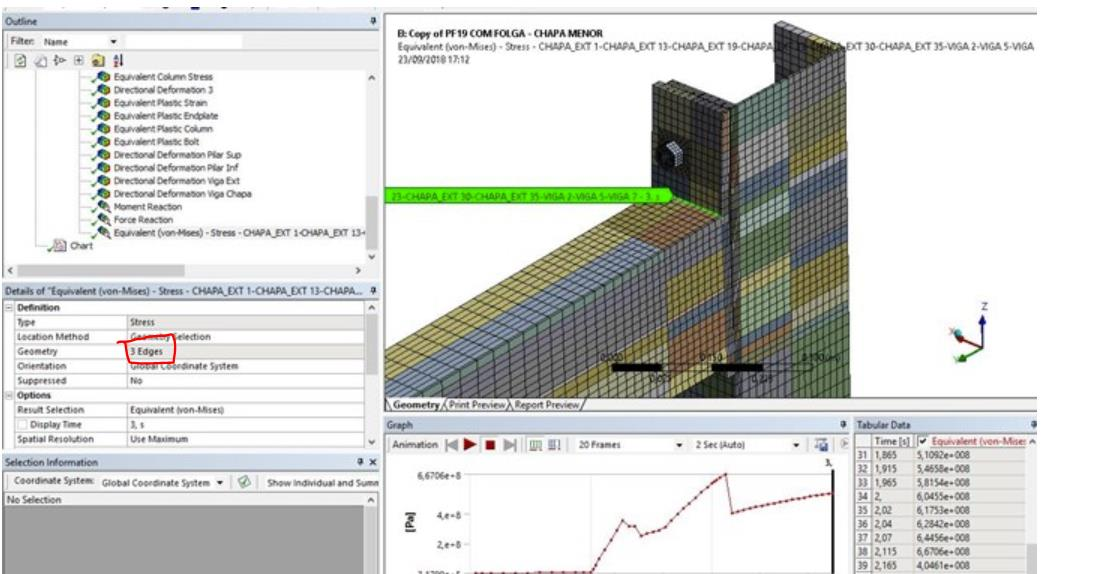Best regards,
Rohith

• Hi all!

I am sorry for not opening a new thread (neither uploading a pic) but for some reason it is impossible since a few days ("Sorry an error occured"). Could you please help me to plot a stress in a function of edge length along a non-linear 2D edge? I need it to compare several simulation with different loads. I am guessing that I might need to get all the values on the nodes with the apdl commands but I don't want to search blindly. Could you please give me any hint?